# 滑动窗口算法（Sliding Window Algorithm）

Sliding window algorithm is used to perform required operation on specific window size of given large buffer or array.

This technique shows how a nested for loop in few problems can be converted to single for loop and hence reducing the time complexity.

# 基本示例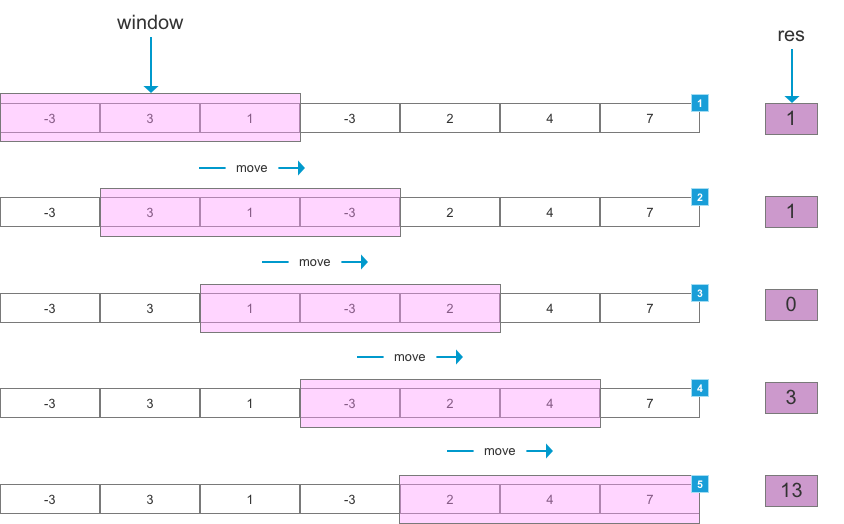# 滑动窗口法的大体框架

• 滑动：说明这个窗口是移动的，也就是移动是按照一定方向来的。

• 窗口：窗口大小并不是固定的，可以不断扩容直到满足一定的条件；也可以不断缩小，直到找到一个满足条件的最小窗口；当然也可以是固定大小。

1. 我们在字符串 S 中使用双指针中的左右指针技巧，初始化 left = right = 0，把索引闭区间 [left, right] 称为一个「窗口」。

2. 我们先不断地增加 right 指针扩大窗口 [left, right]，直到窗口中的字符串符合要求（包含了 T 中的所有字符）。

3. 此时，我们停止增加 right，转而不断增加 left 指针缩小窗口 [left, right]，直到窗口中的字符串不再符合要求（不包含 T 中的所有字符了）。同时，每次增加 left，我们都要更新一轮结果。

4. 重复第 2 和第 3 步，直到 right 到达字符串 S 的尽头。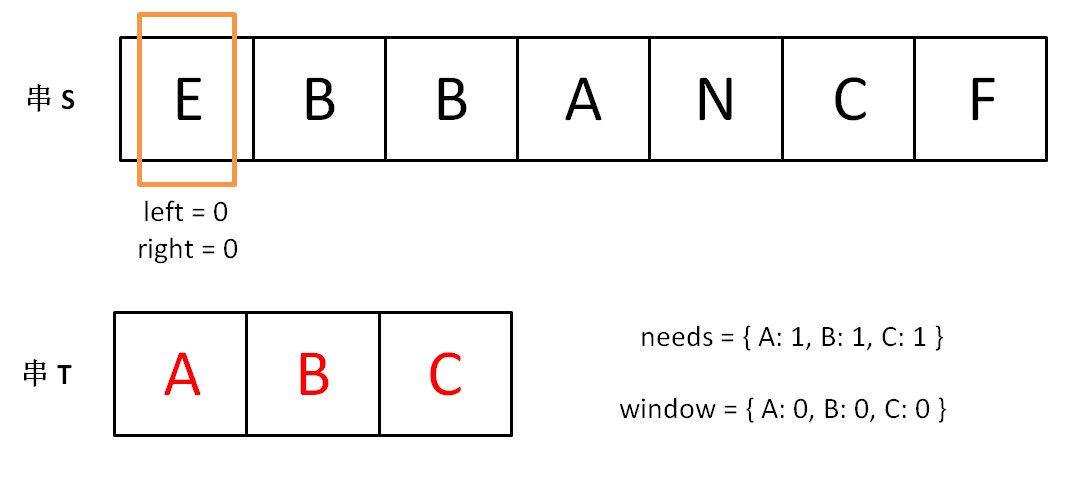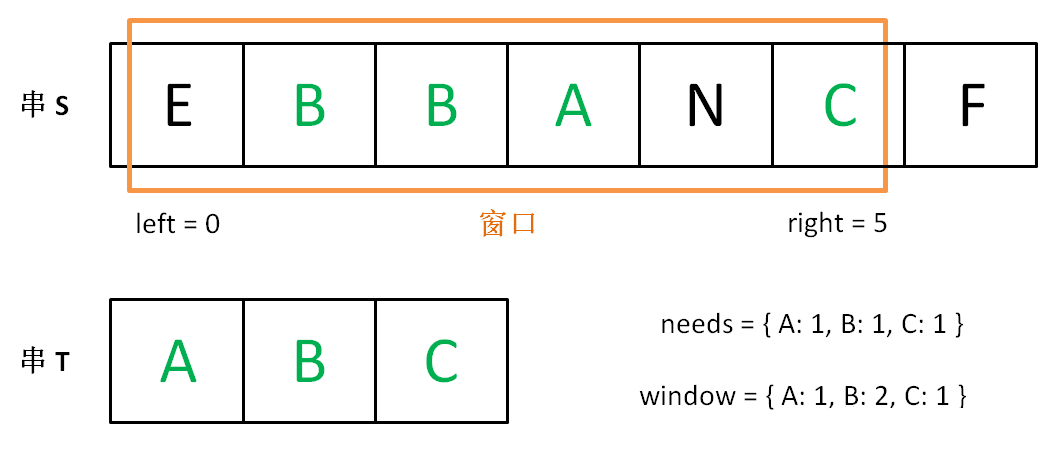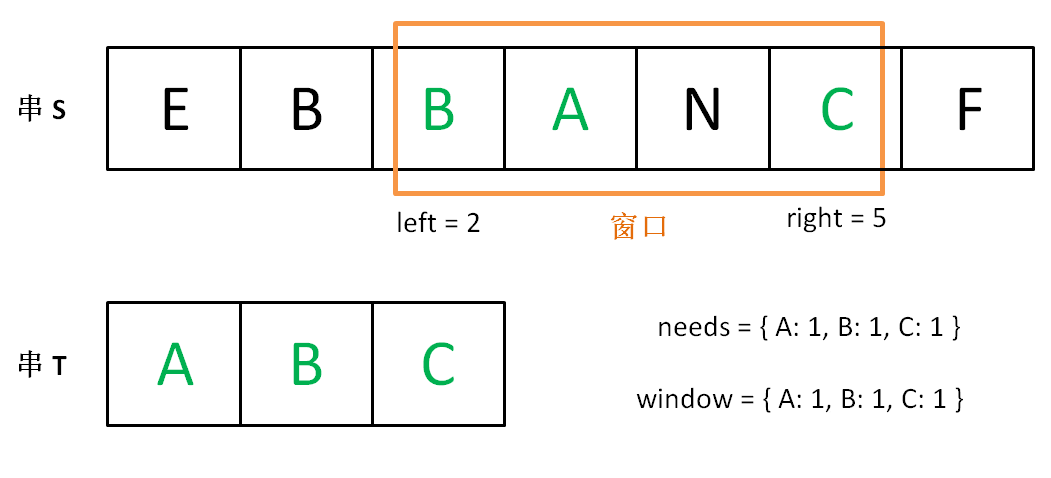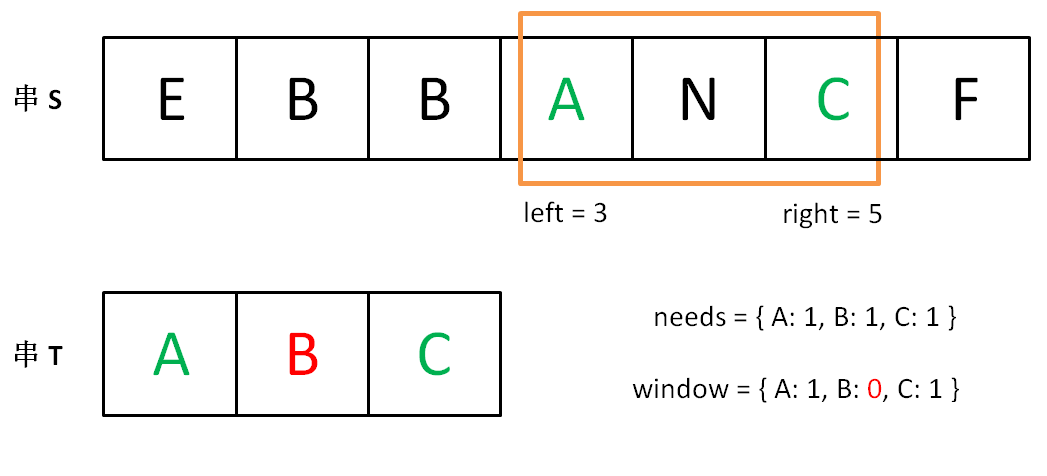string s, t;
// 在 s 中寻找 t 的「最小覆盖子串」
int left = 0, right = 0;
string res = s;

while(right < s.size()) {
right++;
// 如果符合要求，说明窗口构造完成，移动 left 缩小窗口
while (window 符合要求) {
// 如果这个窗口的子串更短，则更新 res
res = minLen(res, window);
window.remove(s[left]);
left++;
}
}
return res;

   // 固定窗口大小为 k
string s;
// 在 s 中寻找窗口大小为 k 时的所包含最大元音字母个数
int  right = 0;while(right < s.size()) {
right++;
// 如果符合要求，说明窗口构造完成，
if (right>=k) {
// 这是已经是一个窗口了，根据条件做一些事情
// ... 可以计算窗口最大值等
// 最后不要忘记把 right -k 位置元素从窗口里面移除
}
}
return res;    

# 算法实例

## 1208. 尽可能使字符串相等

输入：s = "abcd", t = "bcdf", cost = 3

输入：s = "abcd", t = "cdef", cost = 3

输入：s = "abcd", t = "acde", cost = 0

### 代码

class Solution {
public int equalSubstring(String s, String t, int maxCost) {
int left = 0, right =0;
int sum = 0;
int res = 0;　　　　 // 构造窗口
while (right < s.length()) {
sum += Math.abs(s.charAt(right) - t.charAt(right));
right++;　　　　　　　// 窗口构造完成，这时候要根据条件当前的窗口调整窗口大小
while (sum > maxCost) {
sum -=  Math.abs(s.charAt(left) - t.charAt(left));
left++;
}　　　　　　　// 记录此时窗口的大小
res = Math.max(res, right -left);
}
return res;
}
}

## 239. 滑动窗口最大值

输入: nums = [1,3,-1,-3,5,3,6,7], 和 k = 3

滑动窗口的位置                最大值
---------------               -----
[1  3  -1] -3  5  3  6  7       3
1 [3  -1  -3] 5  3  6  7       3
1  3 [-1  -3  5] 3  6  7       5
1  3  -1 [-3  5  3] 6  7       5
1  3  -1  -3 [5  3  6] 7       6
1  3  -1  -3  5 [3  6  7]      7

1 <= nums.length <= 10^5
-10^4 <= nums[i] <= 10^4
1 <= k <= nums.length

### 解答：

class Solution {
public static int[] maxSlidingWindow(int[] nums, int k) {
int right =0;
int[] res = new int[nums.length -k +1];
int index=0;
while (right < nums.length) {　　　　　　　// 这里的list的首位必须是窗口中最大的那位
while (!list.isEmpty() && nums[right] > list.peekLast()) {
list.removeLast();
}　　　　　　　// 不断添加
right++;　　　　　　　// 构造窗口完成，这时候需要根据条件做一些操作
if (right >= k){
res[index++]=list.peekFirst();　　　　　　　　　　// 如果发现第一个已经在窗口外面了，就移除
if(list.peekFirst() == nums[right-k]) {
list.removeFirst();
}
}
}
return res;
}
} 

## 1456. 定长子串中元音的最大数目

输入：s = "abciiidef", k = 3

输入：s = "aeiou", k = 2

输入：s = "leetcode", k = 3

输入：s = "rhythms", k = 4

输入：s = "tryhard", k = 4

1 <= s.length <= 10^5
s 由小写英文字母组成
1 <= k <= s.length

### 解答

class Solution {
public int maxVowels(String s, int k) {
int right =0;
int sum = 0;
int max = 0;
while (right < s.length()) {
sum += isYuan(s.charAt(right)) ;
right++;
if (right >=k) {
max = Math.max(max, sum);
sum -= isYuan(s.charAt(right-k));
}
}
return max;
}

public int isYuan(char s) {
return s=='a' || s=='e' ||s=='i' ||s=='o' ||s=='u' ? 1:0;
}
}

### 算法与数据结构（一）：滑动窗口法总结

posted @ 2020-08-16 17:38  huansky  阅读(52101)  评论(6编辑  收藏  举报